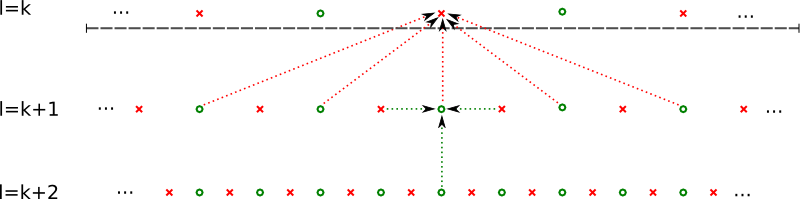SG++-Doxygen-Documentation

Implements the upGradient Method needed for the Laplace operator on prewavelet grids. More...

#include <LaplaceUpGradientPrewavelet.hpp>

## Public Member Functions

Constructor. More...

void operator() (sgpp::base::DataVector &source, sgpp::base::DataVector &result, grid_iterator &index, size_t dim)
This operations performs the calculation of upGradient in the direction of dimension dim More...

Destructor. More...

## Protected Types

typedef sgpp::base::GridStorage::grid_iterator grid_iterator

## Protected Attributes

sgpp::base::GridStoragestorage
Pointer to sgpp::base::GridStorage object. More...

## Detailed Description

Implements the upGradient Method needed for the Laplace operator on prewavelet grids.

The calculation is done iterative and utilizes the following temp variables:

$t_{k,j}=-\frac{6}{10}u_{k,j\pm1}+t_{k+1,2j}\qquad(k,j)\notin G_{n}^{1}$

The correct values are then calculated as follows:

\begin{eqnarray*} r_{k,j}&=&\frac{1}{h_{k}}\left(2t_{k+1,2j}-t_{k+1,2(j\pm1)}\right)\\&&-\frac{6}{10}\frac{1}{h_{k}}\left(-t_{k+1,2(j\pm2)}+2t_{k+1,2(j\pm1)}-2t_{k+1,2j}\right)\\&&+\frac{1}{10}\frac{1}{h_{k}}\left(-t_{k+1,2(j\pm1)}+2t_{k+1,2(j\pm2)}-t_{k+1,2(j\pm2)}\right) \end{eqnarray*}

In case of borders:

\begin{eqnarray*} r_{k,j}&=&\frac{9}{10}\frac{1}{h_{k}}\left(2t_{k+1,2j}-t_{k+1,2(j\pm1)}\right)\\&&-\frac{6}{10}\frac{1}{h_{k}}\left(-t_{k+1,2(j\pm2)}+2t_{k+1,2(j\pm1)}-t_{k+1,2j}\right)\\&&+\frac{1}{10}\frac{1}{h_{k}}\left(-t_{k+1,2(j\pm1)}+2t_{k+1,2(j\pm2)}-t_{k+1,2(j\pm2)}\right) \end{eqnarray*}

Please note, that all values of gridpoints outside of the sparse grid are treated as 0. The following picture depicts all involved grid points and temp values in order to calculate a specific point:All involved gridpoint for the up algorithm (red) and temp points

between grid points (green). The gray line indicates the support of the prewavelet."

## ◆ grid_iterator

 protected

## Constructor & Destructor Documentation

explicit

Constructor.

Parameters
 storage the grid's sgpp::base::GridStorage object

Destructor.

## ◆ operator()()

 void sgpp::pde::LaplaceUpGradientPrewavelet::operator() ( sgpp::base::DataVector & source, sgpp::base::DataVector & result, grid_iterator & index, size_t dim )

This operations performs the calculation of upGradient in the direction of dimension dim

Parameters
 source sgpp::base::DataVector that contains the gridpoint's coefficients (values from the vector of the laplace operation) result sgpp::base::DataVector that contains the result of the down operation index a iterator object of the grid dim current fixed dimension of the 'execution direction'